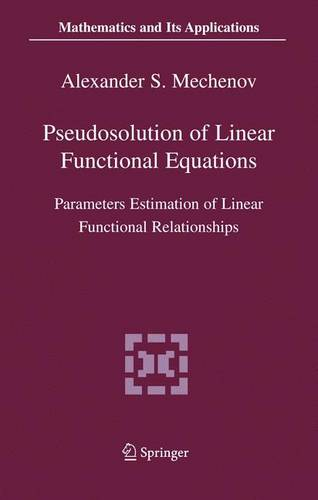•# Pseudosolution of Linear Functional Equations: Parameters Estimation of Linear Functional Relationships - Mathematics and Its Applications 576 (Paperback)

(author)
£69.99
Paperback 238 Pages / Published: 21/03/2005
• We can order this

Usually dispatched within 3 weeks

• This item has been added to your basket
In the book there are introduced models and methods of construction of pseudo-solutions for the well-posed and ill-posed linear functional equations circumscribing models passive, active and complicated experiments. Two types of the functional equations are considered: systems of the linear algebraic equations and linear integral equations. Methods of construction of pseudos6lutions are developed in the presence of passive right-hand side errors for two types of operator errors: passive measurements and active representation errors of the operator, and all their combinations. For the determined and stochastic models of passive experiments the method of the least distances of construction of pseudosolutions is created, the maximum likelihood method of construction of pseudosolutions is applied for active experiments, and then methods for combinations of models of regression, of passive and of active experiments are created. We have constructed regularized variants of these methods for systems of the linear algebraic equations with the degenerated matrices and for linear integral equations of the first kind. In pure mathematics, the solution techniques of the functional equations with exact input data more often are studied. In applied mathematics, problem consists in construction of pseudosolutions, that is, solution of the hctional equations with perturbed input data. Such problem in many cases is incomparably more complicated. The book is devoted to a problem of construction of a pseudosolution (the problem of a parameter estimation) in the following fundamental sections of applied mathematics: confluent models passive, active and the every possible mixed experiments.

Publisher: Springer-Verlag New York Inc.
ISBN: 9780387245058
Number of pages: 238
Weight: 1180 g
Dimensions: 235 x 155 x 15 mm
Edition: 2005 ed.

MEDIA REVIEWS

From the reviews of the first edition:

"This book presents a method of two-stage maximization of a likelihood function, which helps to solve a series of non-solved before well-posed and ill-posed problems of pseudosolution computing systems of linear algebraic equations ... . This book is intended for students, postgraduate students, scientists, and other researchers handling economical and technical data. It is especially intended for those who constantly use regression analysis in their own research and for those who create mathematical software for computers." (Yuehua Wu, Zentralblatt MATH, Vol. 1077, 2006)

## Reviews

Sign In To Write A Review

Please sign in to write a review

Your review has been submitted successfully.